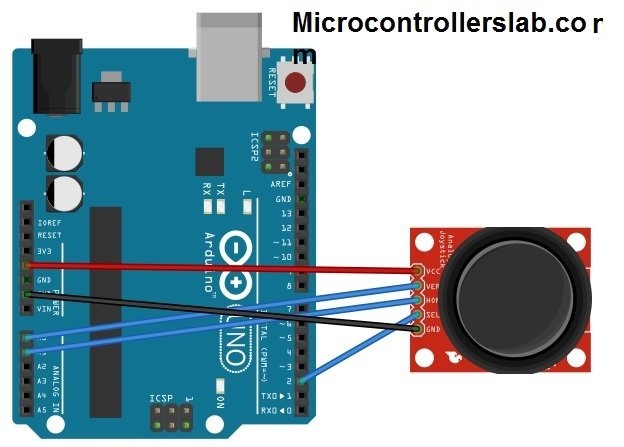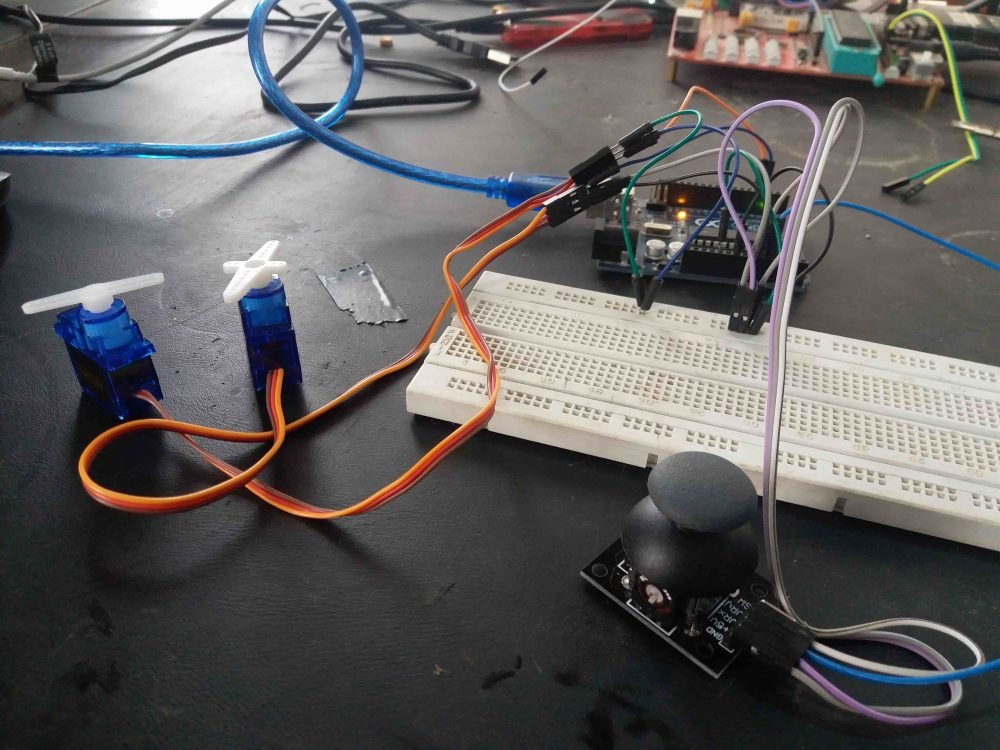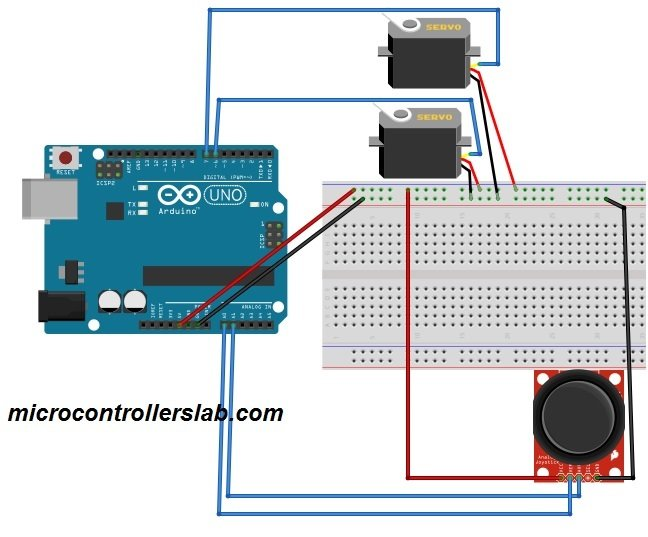# joystick based servo motor control using arduino

Joystick Module for Arduino: This tutorial is on joystick based servo motor control .  In this joystick based servo motor control tutorial, we will first learn how to use joystick module and how to interface it with Arduino and then we will see the example of joystick based servo motor control by using two servo motors.  The joystick module is the simplest to use module and it adds greater flexibility in your projects. It can be used with many microcontrollers but we are using Arduino. Analog Joysticks return analog values. These are basically potentiometers. These values vary from 0 to 1023. When the joystick will be in resting position, then the analog value will be 512. The Arduino analog pins will read the data from the horizontal and vertical pins and the digital pin will be used to read the button. The joystick module has 5 pins, which are

• Supply voltage ( VCC)
• Ground
• X key (Horizontal key).
• Y key (Vertical Key)

There is also a “press to select” push button which can be activated by pressing the joystick down. These can be different on your module depending on from where you buy it. The joystick we are using is analog and it provides more accurate readings then the simple directional joysticks. To design joystick based servo motor control project, you should know how to use joystick module and how to interface servo motor with Arduino. you may also like to check:

### Connection of joystick module with arduino

The connection scheme is very easy. Connect the Arduino 5 v to the VCC of the joystick. Then connect the A0 and A1 to the VER and HOR of the joystick. In last connect the pin 2 of Arduino to SEL of joystick and GND of Arduino to GND of joystick.Arduino                               Joystick

A0                                           VER

A1                                           HOR

Pin 2                                      SEL

GND                                      GND

### Code of joystick interfacing with arduino

// Code for joystick module

```int x_key = A1 ;                                                 // initializing A1 for storing the joystick’s x key value

int y_key = A0 ;                                                 // initializing A0 for storing the joystick’s y key value

int Push_Button= 2 ;                                       // initializing digital pin 2 for joystick’s press to select button

int Horizontal_Position = 0 ;                         // Declaring a variable for storing the value

int y_Position = 0 ;                                           // Declaring a variable for storing the value

int button_State = 0 ;                                     // Declaring a variable for storing the value

void setup ( ) {

Serial.begin ( 9600 ) ;                                    // initializing serial communications at 9600 bps:

pinMode ( x_key, INPUT ) ;                       // Selecting Arduino analog A1 pin as input

pinMode ( y_key, INPUT ) ;                       // Selecting Arduino analog A0 pin as input

pinMode (Push_Button, INPUT_PULLUP ) ;     // This will activate pull-up resistor on the push-button pin

// If you have Arduino version previous to Arduino 1.0.1, then use these commands for activating pull-up resistor.

// pinMode ( button_Pin , INPUT ) ;

// digitalWrite ( button_Pin , HIGH ) ;

}

void loop ( ) {

Horizontal_Position = analogRead ( x_key ) ;  // Reading the value from A1 and storing in Horizontal_Position

y_Position = analogRead ( y_key ) ;                       // Reading the value from A0 and storing in y_Position

button_State = digitalRead ( Push_Button) ;     // Reading the digital pin 2 is high or low

Serial.print ( " Value of X key is " ) ;                        // Printing “ Value of X key is ” on the disply

Serial.print ( Horizontal_Position ) ;                        // Printing value of x key on the display

Serial.print ( " | Value of Y key is " ) ;                     // Printing “ Value of Y key is ” on the screen

Serial.print ( y_Position ) ;                                   // Printing value of y key on the display

Serial.print ( " | Button State is " ) ;                        // Printing “ Button state ” on the display

Serial.println ( button_State ) ;                               // Printing the button is high or low on the display

delay ( 1000 ) ;                                                 // This will add delay between readings

}```

### joystick based servo motor control using Arduino with two servo motorsjoystick based servo motor control  circuit diagram and actual implemented are both shown above and below. For joystick based servo motor control, you need to know how to interface servo motor with Arduino, if you don’t know I recommend you to check following article first:

So now we will see how we can control two servo motors with the help of joystick module. We are using two servo motors. One servo motor can be controlled with x-axis of joystick module and other servo motor is being controlled with y-axis of joystick module. In this example, we will control 2 servo motors using a joystick. This example is used in many projects like game controllers and electronics toy cars.### Components Required for servo motor control with joystick

The components required are the following

• 2 servos
• Joystick
• Arduino uno (you can use any)

The servo motor has three connections which are

• Positive
• Negative
• Signal

The connections are very easier. Connect the servos and joystick as shown in the figure.

### Code of joystick based servo motor control

For this code you will first have to download the library of servo.

```// Controlling 2 Servos Using a Joystick.

#include < Servo.h >                       // including the library of servo motor

int First_Signal_Pin = 6 ;                // initializing pin 6 for first servo

int Second_Signal_Pin =  7 ;         // initializing pin 7 for first servo

int Horizontal_Joystick_Pin = A0 ;  // initializing A0 for horizontal movement

int Vertical_Joystick_Pin = A1 ;  // initializing A1 for vertical movement

// initializing the min and max values for horizontal and vertical movement

int Horizontal_Min = 0 ;

int Horizontal_Max = 180 ;

int Vertical_Min = 0 ;

int Vertical_Max = 180 ;

Servo First_Servo ;

Servo Second_Servo ;

// Declaring variables for storing values

int Horizontal_Value ;

int Horizontal_Servo_Value ;

int Vertical_Value ;

int Vertical_Servo_Value ;

void setup ( )

{

First_Servo.attach ( First_Signal_Pin ) ;                                // Enabling pin 6 for first servo

Second_Servo.attach ( Second_Signal_Pin ) ;                    // Enabling pin 7 for first servo

}

void loop ( )

{

Horizontal_Value  = analogRead ( Horizonta_Joystick_Pin ) ;  // Reading the value from A0

Vertical_Value  = analogRead ( Vertical_Joystick_Pin ) ;    // Reading the value from A1

// Mapping the values for horizontal and vertical movement of joystick.

Horizontal_Servo_Value  = map ( Horizontal_Value, 0 , 1023, ServoH_Min , ServoH_Max ) ;

Vertical_Servo_Value  = map ( Vertical_Value, 0, 1023, ServoH_Min , ServoH_Max ) ;

// Moving the servos

First_Servo.write ( Horizontal_Servo_Value ) ;

Second_Servo.write ( Vertical_Servo_Value ) ;

delay ( 2000 ) ;    // Delay of 2 seconds

}```

As you can see it is very easy to use joystick with arduino and servo motor control code and circuit diagram using Arduino uno R3.

### Video of joystick based servo motor control

For demonstration check the video

If you have any issue after reading servo motor control with joystick article. I recommend you to comment on this post.

### 3 thoughts on “joystick based servo motor control using arduino”

1. hi, if i would like to control 4 servomotors with 2 joysticks with one arduino board. how i can do it?